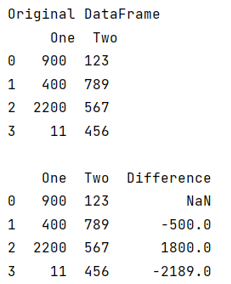# Adding a column that result of difference in consecutive rows in Pandas

Given a Pandas DataFrame, we have to add a column that result of difference in consecutive rows in Pandas? By Pranit Sharma Last updated : September 24, 2023

Pandas is a special tool that allows us to perform complex manipulations of data effectively and efficiently. Inside pandas, we mostly deal with a dataset in the form of DataFrame. DataFrames are 2-dimensional data structures in pandas. DataFrames consist of rows, columns, and data.

## Problem statement

Given a Pandas DataFrame, we have to add a column that result of difference in consecutive rows in Pandas.

## Adding a column that result of difference in consecutive rows in Pandas

To achieve this task we will first create a DataFrame and then we will use pandas.DataFrame.diff() method. This method is used to get the difference between the row values. If we do not specify any parameter then by default it returns the difference of the previous row.

The syntax of DataFrame.diff() method:

```DataFrame.diff(periods=1, axis=0)
```

The parameter(s) of DataFrame.diff() method:

• axis: It represents the axis to check the difference
• periods: It represents which row/column differences have to be calculated
Note

To work with pandas, we need to import pandas package first, below is the syntax:

```import pandas as pd
```

Let us understand with the help of an example,

## Python program to add a column that result of difference in consecutive rows in Pandas

```# Importing pandas package
import pandas as pd

# Creating a dictionary
d = {
"One": [900, 400, 2200, 11],
"Two": [123, 789, 567, 456]
}

# Creating a DataFrame
df = pd.DataFrame(d)

# Display original DataFrame
print("Original DataFrame\n",df,"\n")

# Calculating difference
df["Difference"] = df["One"].diff()
print(df)
```

### Output

The output of the above program is: